American Journal of Chemical Engineering
Volume 4, Issue 1, January 2016, Pages: 16-22

Modelling and Simulation of Gauze Reactor of Ammonia Oxidation

Mohamed Abdel Salam1, *, Ehssan Nassef2, Eman Elkheriany1, Yehia El Tawel1

1Chemical Engineering Department, Faculty of Engineering, Alexandria University, Alexandria, Egypt

2Petrochemicals Engineering Department, Faculty of Engineering, Pharos University, Alexandria, Egypt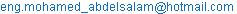(M. A. Salam)

Mohamed Abdel Salam, Ehssan Nassef, Eman Elkheriany, Yehia El Tawel. Modelling and Simulation of Gauze Reactor of Ammonia Oxidation. American Journal of Chemical Engineering. Vol. 4, No. 1, 2016, pp. 16-22. doi: 10.11648/j.ajche.20160401.13

Abstract: Modelling of catalytic ammonia oxidation reactor for nitric oxide production in nitric acid plant over gauzes of Pt/Rh catalyst was the subject of current study. Viscosity, mass transfer and kinetic equations were used for the gas mixture feeding the reactor, partial pressure and mole fraction of ammonia was essential, also wire diameter was considered to detect platinum losses during oxidation operation. In order to investigate the performance of catalytic oxidation reactor, ordinary equation for determining of conversion yields of ammonia to nitric oxide was solved in mathematical model numerically. Variations of conversion with inlet mixture temperature, mixture concentration, inlet pressure and gauze temperature during cycle were investigated. Platinum losses rate as platinum oxide (PtO2) was studied with gauzes surface temperature by using numerical solution of the mathematical model. Modelling results were compared with an industrial reactor data. Comparing industrial data for all studied variables with those obtained by using mathematical model have shown the higher degree of accuracy of the model to predict the operating values with minimum deviation.

Keywords: Nitric Acid, Kinetics of Ammonia Oxidation, Platinum Losses

1. Introduction

To be able to analyze and optimize the operation of a plant, one must first have a mathematical model describing the real behavior of the process. The modelling of a plant can be done in many different ways; one can use the physical and chemical relationships the process is based on, or one can try to find the model experimentally. So we can have different models for the same process.

Catalytic oxidation of ammonia is commercially one of the most important reactions in heterogeneous catalysis. Uronen  has shown that the overall oxidation rate is controlled by the transfer of NH3 from the bulk gas phase to the platinum surface.  The behavior of an industrial reactor for the oxidation of ammonia with Pt–Rh gauzes was simulated by means of a mathematical model accounting for the axial dispersion of the components, the effect of temperature on the physico-chemical properties of the reacting mixture. The effect of the preheating temperature, the input mixture composition, pressure and reactor load on the NO yield was examined. The calculated NO yields agree well with the values actually attained in industrial reactors. The effect of the reaction mixture composition and total pressure on the yield is also consistent with observed dependences. 

Reactors containing a catalyst in the form of a gauze are applied to the production of nitrogen oxides during the production of nitric acid (the Ostwald process). The reactor for the oxidation of ammonia considered is shown in figure 1. It is composed of: (1) Catalyst layer, (2) Layer for catchment of the escaped catalyst, (3) cooling area. The rapid cooling helps in minimizing the loss from decomposition of generating NO. 

In dependence on the catalyst used and on the process conditions (temperature, pressure, gas phase composition), the catalytic oxidation of NH3 can generally involve the following reactions: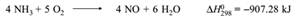(I)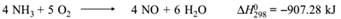(II)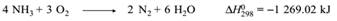(III)

In addition, the following processes also play a minor rule.(IV)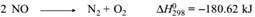(V)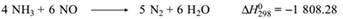(VI)

The total pressure during the combustion of NH3 is the most important technological parameter of industrial oxidation of ammonia. Normally, the working temperature in the catalyst bed is about 890°C, and increases with increasing pressure. 

The ammonia to air ratio is typically 9.5 to 11.5 vol. %, the time of contact with the catalyst is 103 to 10-4 sec. . The platinum catalyst, which is usually an alloy with rhodium (2-10%), has the form of gauzes made up of wires.

The objective of the present work was to find an appropriate mathematical model for a reactor for the oxidation of ammonia on Pt-Rh gauzes. The model should account for the following basic features of the process:

Increase in NO yield if the specific surface area of the catalyst is increased.

Decrease in the reaction selectivity with respect to NO if the total pressure in the reactor is increased.

Decrease in the NO yield if the concentration of NH3 in the feed is increased.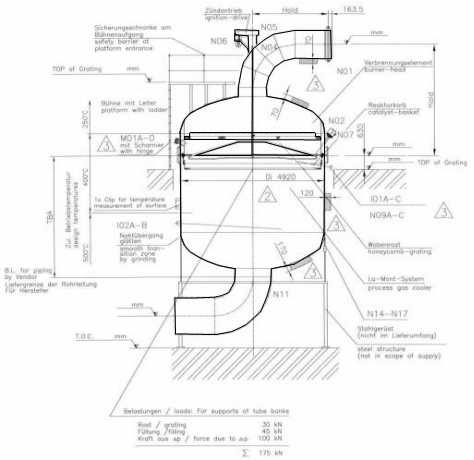Figure 1. Reactor for oxidation of ammonia .

2. Model Development

2.1. Thermodynamics of Ammonia Oxidation

The reaction is highly exothermic and not equilibrium limited. The following relations have been reported for this reaction system in the limited temperature range of interest. 

(-∆H) = [54250 – 0.4(T-298)]*10^-3 Kcal/g mole of NH3 reacted              (1)

Where T in K

Basis equation is:

NH3 + 5/4 O2 → NO+ 3/2 H2    (VII)

Cpm = 6.45 + T (1.52 + 4.08 yA) 10-3      (2)

Where yA is the mole fraction of ammonia in feed. This heat capacity equation applies in the region of T =600-1100 K and yA = 0.09-0.12.

For convenience the equations for viscosity and thermal conductivity of the mixture in the same temperature regions will also be given at this point.

λf = (3.31 + 12.77*10-3 T) 10-4 Kcal K/cm sec     (3)

μf = (12.5 +29.50*10-3 T) 10-5 g/cm sec       (4)

Where T in K

2.2. Kinetics of Ammonia Oxidation

Early experimental work on this process demonstrated that under normal operating conditions the physical transport of ammonia to the catalyst surface is the rate determining step. 

Bases on the physical and chemical phenomena involved, we can qualitatively conclude the most important factors affecting on the conversion of the oxidation process are: ammonia to air ratio in the feed, feed temperature, total gas charge on sieves, plant pressure, number of sieves and sieve dimensions. 

At low temperatures or with deactivated catalyst the chemical rate can become limiting or partially limiting. With active catalyst, it is clear that high temperatures and high velocities will produce essentially total conversion of ammonia. The maximum temperature is limited by the aforementioned catalyst loss that becomes excessive much above 900°C. The loss per unit surface area is apparently a direct function of gauze temperature, mass flow rate, and ammonia/oxygen ratio in the feed. 

On the of mass-transfer control the rate of ammonia oxidation may be written in terms of a mass-transfer coefficient with the ammonia partial pressure at the catalyst surface assumed to be zero for this rapid reaction. 

(-rA) = KgA sawR PA          (5)

Where PA is the partial pressure of ammonia in bulk fluid and awR is the surface area per unit volume of screen. For shallow beds, axial diffusion must surely be important, but the very high flow rates encountered in commercial equipment cause it to be less important than the problem of equal flow distribution across the gauzes. By including a gas distributor below the catalyst pad like honey comb, this problem is greatly alleviated. 

The model is developed based on plug flow. 

(-G/MF) dyA = (-rA) dZ         (6)

Where (-rA) is the g-moles of NH3 converted/cm3 sec.

For this rapid reaction assume that partial pressure of ammonia on the catalyst surface is zero. Then the rate of transfer of ammonia becomes 

(-rA) dZ = Kga saWR yA PdZ = KgA syA Pfw dns    (7)

Where ns is the number of gauzes and fw is the wire area per gauze cross sectional area for one gauze. 

(-G/Mf) dyA = Kga s yA P fw dns         (8)

or integrating and simplifying by assuming constant number of moles 

Kga s P ns fw = (G/Mf) ln (YA1/YA2) = (-G/Mf) ln (1-XA)   (9)

or

ln (1-XA) = -Kga s P ns fw Mf/G         (10)

Where,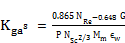(11)

Where Mm is the molecular weight of the mixture.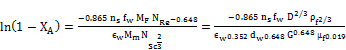(12)

Where dw is the diameter of wire. MF/Mm is approximately unity.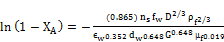(13)

D = 0.227 (T/293)3/2 (1/P) cm2/sec       (14)

M = MF = (32)(0.21)(1-YA) + (28.01)(0.79)(1-YA) + 17.03 yAo = 28.85 + 11.82 YAo     (15)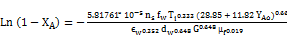(16)

To calculate the rate of Pt losses, 

R = 1.09*1010 (TO2)-1/2 (PO2) Pe exp (-42500/RT)    (17)

In this case, the composition of the catalyst is 95% Pt- 5% Rh. The total weight of the catalyst is 70 Kg. So, the weight of Pt is 66.5 Kg. The weight of Rh is 3.5 Kg. . The effective gauze diameter is 4600 mm, the wire diameter is 0.0076 mm. The operating cycle is six months. 

There are several chemical reactions occurred in parallel with the formation of NO. Table (1) describes the rates of these chemical reactions. The most dominant reactions is described in equations 19, 20.

Table 1. Chemical reaction rates .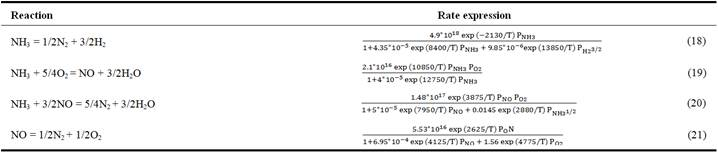3. Results and Discussion

The developed model predictions for catalytic ammonia oxidation reactor were investigated and compared with the industrial data. The figures were simulated using Matlap R2014a.

3.1. Effect of Inlet Mixture Temperature on NO Yield

Figure 2 shows the effect on inlet mixture temperature of mixed gases to burner on NO yield for knitted gauzes. It is clear that NO yield decreases with increasing inlet temperature and this is because of catalytic decomposition of NO at the gauzes and the side reaction of ammonia at the metallic parts of the reactor body before coming in contact with O2. 

The plot demonstrates that model data agrees with industrial data as NO yield decreases with increasing inlet mixture temperature. In calculated results, the NO yield becomes steeper at higher inlet temperatures. In experimental results, the NO yield decreases gradually and at higher inlet temperatures, the NO yield becomes sharp because at higher temperatures, the reaction between NO and NH3 takes place. Minimum deviation is between 460 to 500K. Maximum deviation is between 300 to 360K. These result is similar to those produced by A Farouk .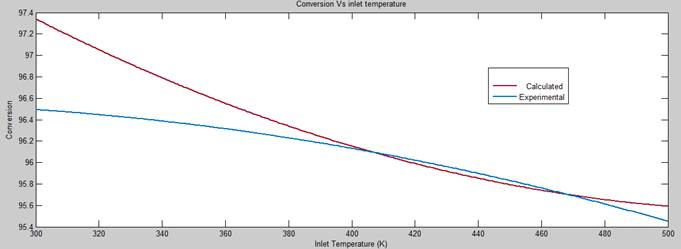Figure 2. Conversion vs inlet temperature.

3.2. Effect of Inlet Mixture Temperature on Rate of Reactions

Figure 3 shows the inlet mixture temperature on rate of reactions. The main two reactions are the reaction of ammonia with oxygen to produce nitric oxide and water, the second one is the reaction of ammonia with oxygen to produce nitrogen and water. The rate of NO reaction increases with increasing inlet temperature according to Arrhenius law as the reaction rate constant increases with increasing temperature. Also, the rate of N2 reaction increases gradually with increasing temperature, and increases sharply at higher temperatures. This is another reason of decreasing NO yield sharply at higher temperatures.

3.3. Effect of Inlet Pressure on Rate of Reactions

Figure 4 shows the effect of inlet pressure on rate of reactions. The rate of reaction to produce NO increases sharply with inlet pressure, but increases steeply at higher pressure. The rate of reaction to produce N2 increases gradually with inlet pressure.

It is supposed that the NO yield will decrease with increasing inlet pressure as the rate of reaction to produce NO becomes steeper at higher pressure while the rate of reaction to produce N2 increases gradually.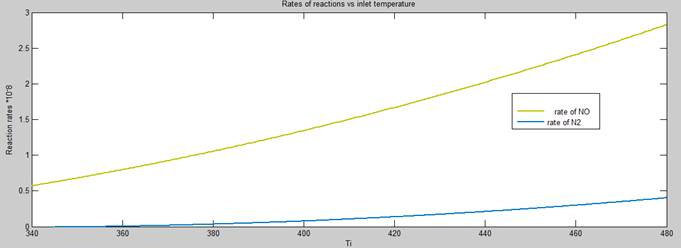Figure 3. Reaction rates vs inlet temperature.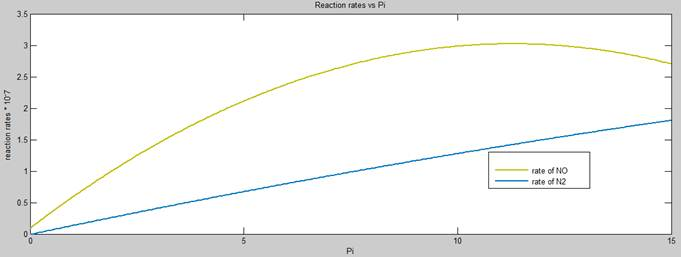Figure 4. Reaction rates vs ammonia pressure.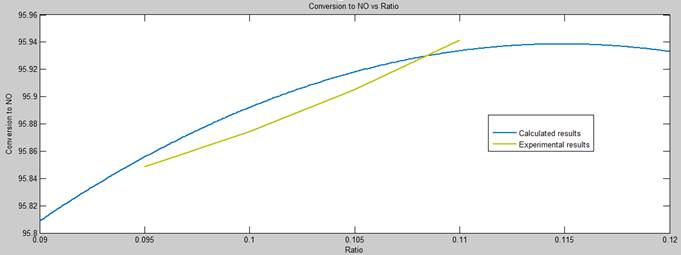Figure 5. Plot of conversion vs ratio.

3.4. Effect of inlet Ratio on NO Yield

Figure 5 shows the effect of inlet ammonia/air ratio on NO yield. The calculated results shows that the NO yield increases gradually till ammonia/air ratio becomes 11.5%, then NO yield decreases gradually. The experimental results shows that before ammonia/air ratio becomes 11.5%, the NO yield increases gradually with increasing ammonia/air ratio. The experimental results can’t be collected at ammonia/air ratio higher than 11.5% as the plant will be automatically shut down to safe zone to keep the ammonia/air ratio away from explosive limit. 0.1% increase in ammonia/air ratio increases gauze temperature by 6.7°C. 

3.5. Effect of Gauze Temperature on NO Yield

Figure 6 shows the calculated results of the model showing the effect of gauze temperature on NO yield. The NO yield increases gradually with increasing gauze temperature.

Figure 7 differentiate between the calculated results from the mathematical model and the experimental results. As the gauze temperature increases, the NO yield also increases.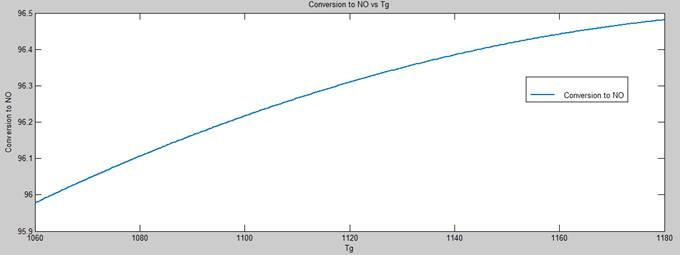Figure 6. Plot of NO conversion vs gauze temperature (model data).Figure 7. Plot of NO conversion vs gauze temperature.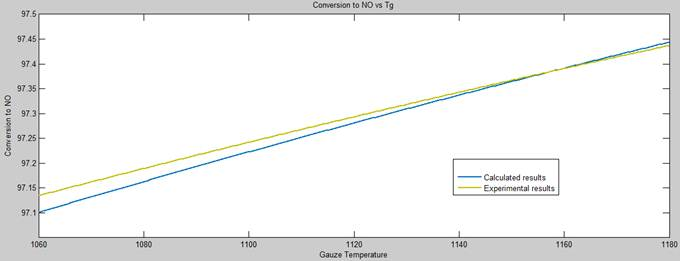Figure 8. Plot of NO conversion vs gauze temperature.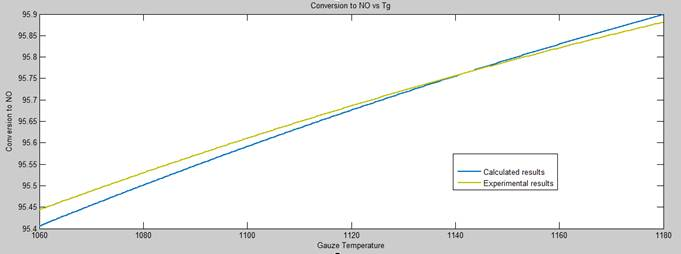Figure 9. Conversion vs gauze temperature.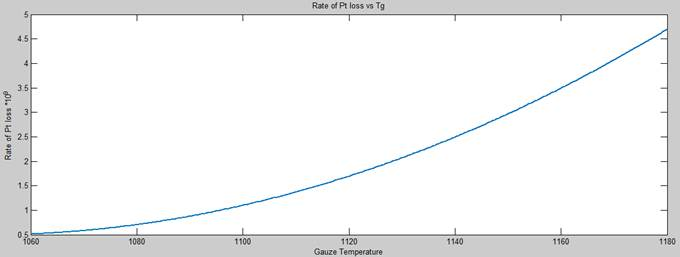Figure 10. Rate of Pt losses vs gauze temperature.

3.6. Effect of Gauze Temperature on Rate of Pt Losses

Figure 10 shows the calculated results of the correlation between the rate of Pt losses and the gauze temperature. As the gauze temperature increases, the rate of Pt losses increases. This is the main reason of the limitation on gauze temperature.

The rate of Pt losses increases steeper with increasing gauze temperature, while it increases sharply with higher gauze temperatures.

4. Conclusion

From the results of the present work, it can be concluded the following:

1. For inlet mixture temperature, the model data showed that as the inlet mixture temperature increases, the rate of production of N2 increases in a rate faster than rate of production of NO. Also, the model data showed that NO yield decreases with increasing inlet mixture temperature which agreed with industrial data for knitted gauzes.

2. For inlet mixture pressure, the model data showed that with increasing pressure, the rate of production of N2 increases in a faster rate than rate of production of NO. The model also showed that with increasing the pressure of inlet mixture, the NO yield will decrease.

3. For ammonia to air ratio, Model data showed that NO yield increases with increasing ratio and when the ratio become 11.2%, the NO yield will decrease. The industrial data confirmed only till 11% ratio as it is forbidden to operate the plant at ratio more than 11% to be away enough from lower explosive limit.

4. For gauze temperature, the model data showed that with increasing gauze temperature, the NO yield will increase. The industrial data confirmed that issue. But, with increasing gauze temperature, rate of Pt losses will also increase. This is why there is a limitation on increasing of gauze temperature.

List of Abbreviations

∆H: change in enthalpy (Kcal)

CPm: Specific heat of mixture (Kcal/g.K)

YA: mole fraction of A in mixture

λf: Thermal conductivity (Kcal.K/cm.sec)

μf: Viscosity (g/cm.sec)

(-rA): rate of reaction (mole/sec)

KgAs: Mass transfer coefficient (cm/sec)

aWR: Surface area per unit volume (cm2/cm3)

PA: Partial pressure of A (atm)

G: Mass velocity (g/cm2 sec)

Mf: Molecular weight of feed gas (g-mole)

fw: Wire area per gauze cross-sectional area for one gauze

ns: Number of gauzes

XA: Mole fraction of converted ammonia to NO

NRe: Reynolds number

NSc: Schmidt number

ϵw: Porosity factor

Ti: mean film temperature (K)

References

1. P. Uronen, E. Kiukaanniemi, "Optimization of the ammonia oxidation process used in the manufacture of nitric acid", University of Oulu, Finland.
2. Vlastimil FILA and Bohumil BERNAUER "A mathematical model of a gauze reactor for the ammonia oxidation", Department of Inorganic Technology, Prague Institute of Chemical Technology, The Czech Republic 1993.
3. HOWARD F. RASE, Chemical reactor design for process plants, Case studies and design data, University of Texas at Austin.
4. Kirk/Othmer, "Encyclopedia of Chemical Technology" 3rd edition, John Wiley & Sons, New York, (1999-2014).
5. E. J. Nowak, "Catalytic oxidation of ammonia", Process Research Division, New Jersey, 1966.
6. "UHDE Nitric acid plant manual", EHC Company, Egypt, 2013.
7. Daniel A. Hickman and Lanny D. Schmidt, "Modeling Catalytic Gauze Reactors: Ammonia oxidation" Department of Chemical Engineering and Materials Science, University of Minnesota, Minneapolis, Minnesota, 1991.
8. A. Farouk, H. Farrag, "Modelling of ammonia burner" Alexandria University, 2010.
9. Rdzawski, Z. M. and Stobrawa, J. P., "Microstructure stability of the Pt/Rh alloys used for catalytic ammonia oxidation", Journal of achievements in materials and manufacturing engineering, 24, (2007).
10. Chatteree, I. B. and Joshi, J. B., "Modeling, simulation and optimization: Mono pressure nitric acid process", Chemical Engineering Journal, 138, 556-557, (2008).
11. Lawrie Lloyd, "Handbook of industrial catalysts", springer, 2011.
12. Jens Hagen, "Industrial catalysis a practical approach" Wiley-VCH, 2006.
13. Satterfield C. N, "Heterogeneous Catalysis in Practice" McGraw-Hill, New York 1980.
14. "Technical specification of Ammonia oxidation reactor", UHDE, EHC company, Egypt,2013.

 Contents 1. 2. 2.1. 2.2. 3. 3.1. 3.2. 3.3. 3.4. 3.5. 3.6. 4.
Article ToolsAbstractPDF(2872K)